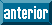## Articulo

•Citado por SciELO
•Accesos

•Similares en SciELO

## versión impresa ISSN 1409-2433

#### Resumen

ROJAS-TORRES, Luis. Robustness of confirmatory factor analysis fit indices to outliers. Rev. Mat [online]. 2020, vol.27, n.2, pp.383-404. ISSN 1409-2433.  http://dx.doi.org/10.15517/rmta.v27i2.33677.

The goal of this work is to evaluate the robustness of several Confirmatory Factor Analysis fit indices (SRMR, RMSEA, TLI, CFI and GFI) to the precense of outliers. For this purpose, it was planed a simulation study with 3 × 4 × 2 conditions: sample size (100, 200 and 500), outliers percentage (0%, 1%, 5% and 10%) and number of variables with outliers (1 and 2). The baseline data sets (0% of outliers) by sample size were simulated from a distribution which fit to a CFA with three factors correlated. Data bases with outliers were created from substitution of observations in baseline data sets. Later, in every data base was estimated a CFA with three factors correlated. It was obtained that all indices with classical cutoffs were robust to outliers with sample sizes of 200 and 500. With 100 observations, it was obtained that fit indexes were robust to outliers, but considering cutoffs adjusted by the factorial structure and the sample size.

Palabras clave : outliers; confirmatory factor analysis; robustness; simulations study; Monte Carlo error..

· resumen en Español     · texto en Español     · Español (pdf )